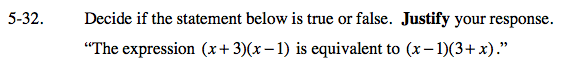Home > CAAC > Chapter 5 > Lesson 5.1.3 > Problem5-32

5-32.

Decide if the statement below is true or false. Justify your response. Homework Help ✎

“The expression (x + 3)(x − 1) is equivalent to (x − 1)(3 + x).”These expressions are equivalent because of the Commutative properties of Addition, x + 3 = 3 + x,
and because of the Commutative properties of Multiplication, (x + 3)(x − 1) = (x − 1)(x + 3).